# Keras深度學習實戰（7）——卷積神經網絡詳解與實現

Keras深度學習實戰（7）——卷積神經網絡詳解與實現

• 0. 前言
• 1. 傳統神經網絡的缺陷
• 1.1 構建傳統神經網絡
• 1.2 傳統神經網絡的缺陷
• 2. 使用 Python 從零開始構建CNN
• 2.1 卷積神經網絡的基本概念
• 2.2 卷積和池化相比全連接網絡的優勢
• 3. 使用 Keras 構建卷積神經網絡
• 3.1 CNN 使用示例
• 3.2 驗證 CNN 輸出
• 4. 構建 CNN 模型識別 MNIST 手寫數字
• 4.1 任務與模型分析
• 4.2 CNN 模型構建與訓練
• 相關鏈接

0. 前言

1. 傳統神經網絡的缺陷

• 建立一個 NN 模型，以預測 MNIST 手寫數字標簽
• 獲取所有標簽為 1 的圖像，並取這些圖像的均值生成新圖像
• 使用構建的 NN 預測在上一步中生成的均值圖像的標簽
• 將均值圖像向左或向右平移若幹個像素，生成新圖像，並使用 NN 模型對生成的新圖像進行預測

1.1 構建傳統神經網絡

1. 加載所需庫和 MNIST 數據集：
from keras.datasets import mnistfrom keras.layers import Flatten, Densefrom keras.models import Sequentialimport matplotlib.pyplot as pltfrom keras.utils import np_utilsimport numpy as np(x_train, y_train), (x_test, y_test) = mnist.load_data()
1. 獲取訓練集中標簽為 1 的數字圖片：
x_train1 = x_train[y_train==1]
1. 將訓練數據整形以符合網絡輸入尺寸要求，並進行數據規范化：
num_pixels = x_train.shape * x_train.shapex_train = x_train.reshape(x_train.shape, num_pixels).astype('float32')x_test = x_test.reshape(x_test.shape, num_pixels).astype('float32')x_train = x_train / 255.x_test = x_test / 255.
1. 對圖像標簽進行獨熱編碼：
y_train = np_utils.to_categorical(y_train)y_test = np_utils.to_categorical(y_test)num_classes = y_train.shape
1. 然後，構建模型並進行擬合：
model = Sequential()model.add(Dense(1024, input_dim=num_pixels, activation='relu'))model.add(Dense(num_classes, activation='softmax'))model.compile(loss='categorical_crossentropy', optimizer='adam', metrics=['acc'])model.fit(x_train, y_train,            validation_data=(x_test, y_test),            epochs=5,            batch_size=1024,            verbose=1)
1. 接下來，使用標簽為 1 的所有圖像的均值生成新圖像，並使用訓練後的模型預測此圖像的標簽。首先，生成圖像：
pic = np.zeros((x_train1.shape, x_train1.shape))pic2 = np.copy(pic)for i in range(x_train1.shape):    pic2 = x_train1[i,:,:]    pic = pic + pic2pic = pic / x_train1.shapeplt.imshow(pic, cmap='gray')plt.show()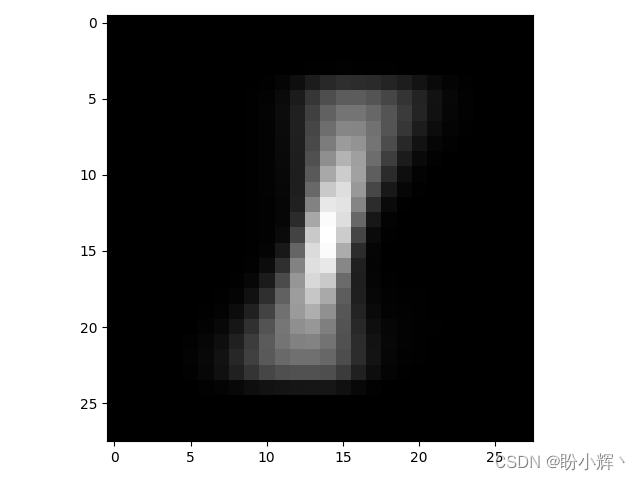1. 最後，查看神經網絡對於此圖像的預測：
p = model.predict(pic.reshape(1, -1)/255.)print(p)c = np.argmax(p)print('神經網絡預測結果：', c)

np.argmax() 函數用於返回一個 Numpy 數組中最大值的索引，在以上示例中，最大值的索引就是模型預測概率最大的類別。以上擬合後的模型，輸出的預測結果如下：

[[8.6497545e-05 9.2336720e-01 2.6006915e-03 5.4899454e-03 4.6638816e-04  8.7751285e-04 6.4074027e-04 2.3328003e-03 6.3134938e-02 1.0033193e-03]]神經網絡預測結果： 1

1.2 傳統神經網絡的缺陷

for i in range(pic.shape):    if i < 20:        pic[:,i]=pic[:,i+1]plt.imshow(pic, cmap='gray')plt.show()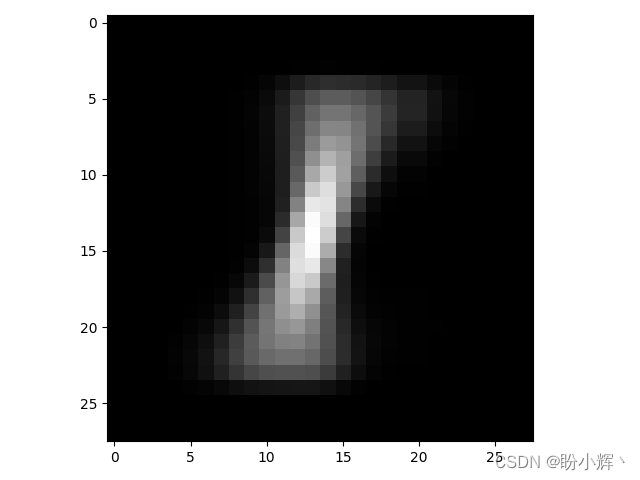p = model.predict(pic.reshape(1, -1)/255.)print(p)c = np.argmax(p)print('神經網絡預測結果：', c)

[[2.3171112e-03 5.3161561e-01 2.6453543e-02 8.1305495e-03 5.2826328e-04  3.4600161e-02 4.3771293e-02 3.4394194e-04 3.5101682e-01 1.2227822e-03]]神經網絡預測結果：1

pic=np.zeros((x_train1.shape, x_train1.shape))pic2=np.copy(pic)for i in range(x_train1.shape):    pic2=x_train1[i,:,:]    pic=pic+pic2pic=(pic/x_train1.shape)pic2=np.copy(pic)for i in range(pic.shape):    if ((i>6) and (i<26)):        pic[:,i]=pic2[:,(i-3)]plt.imshow(pic, cmap='gray')plt.show()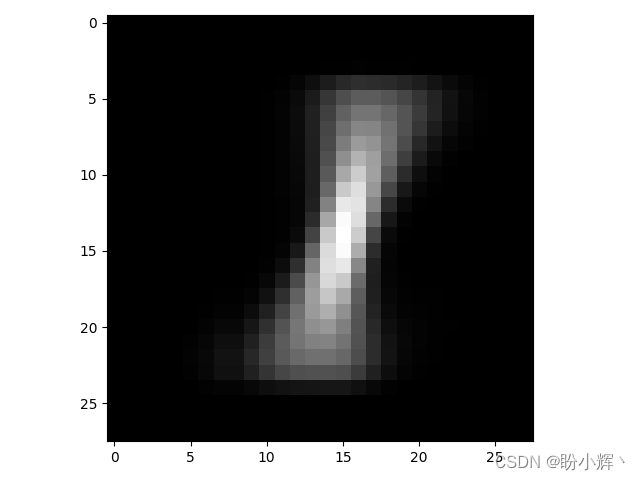p = model.predict(pic.reshape(1, -1)/255.)print(p)c = np.argmax(p)print('神經網絡預測結果：', c)

[[0.00519334 0.0018531  0.07164755 0.33244154 0.3407778  0.00380969  0.00090572 0.19745363 0.0096615  0.03625605]]神經網絡預測結果：4

2. 使用 Python 從零開始構建CNN

2.1 卷積神經網絡的基本概念

2.1.1 卷積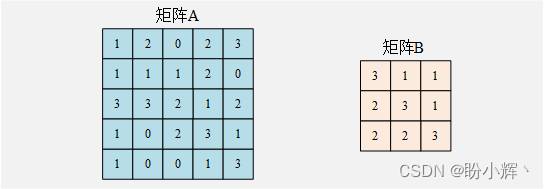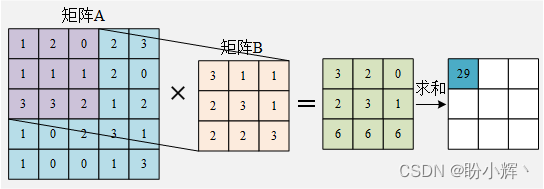1 × 3 + 2 × 1 + 0 × 1 + 1 × 2 + 1 × 3 + 1 × 1 + 3 × 2 + 3 × 2 + 2 × 3 = 29 1\times 3+2\times 1+0\times 1+1\times 2+1\times 3+1\times 1+3\times 2+3\times 2 + 2\times 3=29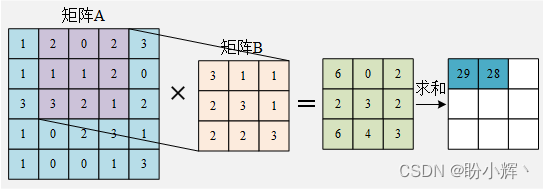2 × 3 + 0 × 1 + 2 × 1 + 1 × 2 + 1 × 3 + 2 × 1 + 3 × 2 + 2 × 2 + 1 × 3 = 28 2\times 3+0\times 1+2\times 1+1\times 2+1\times 3+2\times 1+3\times 2+2\times 2 + 1\times 3=28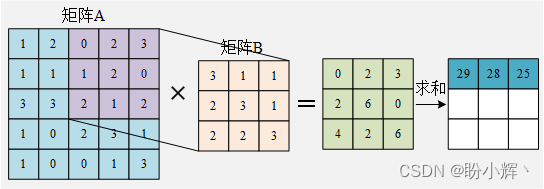0 × 3 + 2 × 1 + 3 × 1 + 1 × 2 + 2 × 3 + 0 × 1 + 2 × 2 + 1 × 2 + 2 × 3 = 25 0\times 3+2\times 1+3\times 1+1\times 2+2\times 3+0\times 1+2\times 2+1\times 2 + 2\times 3=25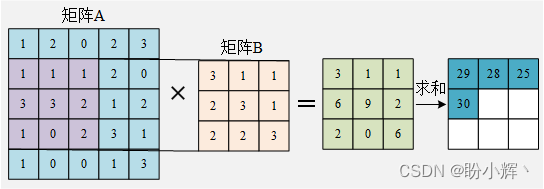1 × 3 + 1 × 1 + 1 × 1 + 3 × 2 + 3 × 3 + 2 × 1 + 1 × 2 + 0 × 2 + 2 × 3 = 30 1\times 3+1\times 1+1\times 1+3\times 2+3\times 3+2\times 1+1\times 2+0\times 2 + 2\times 3=30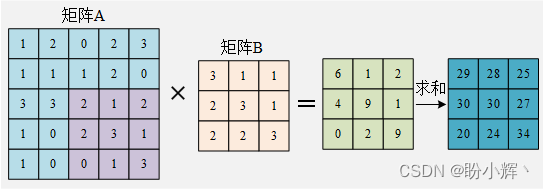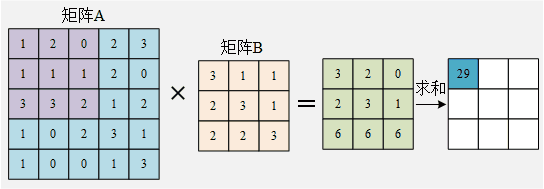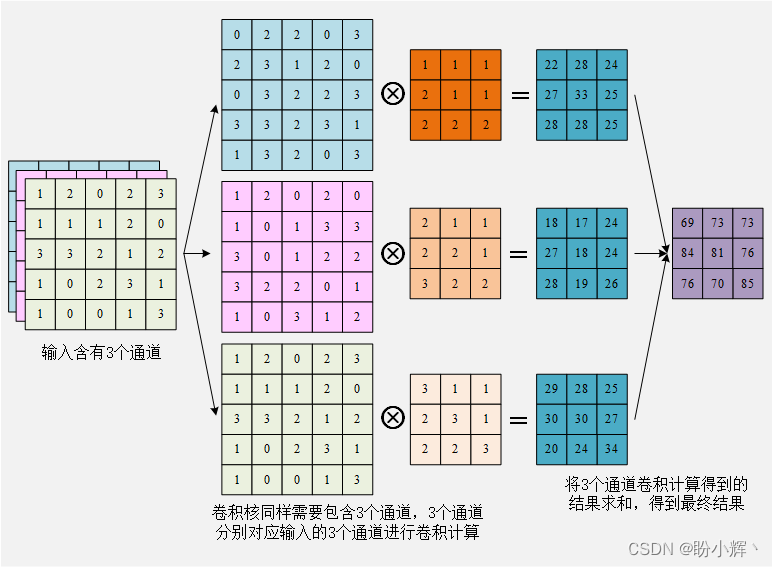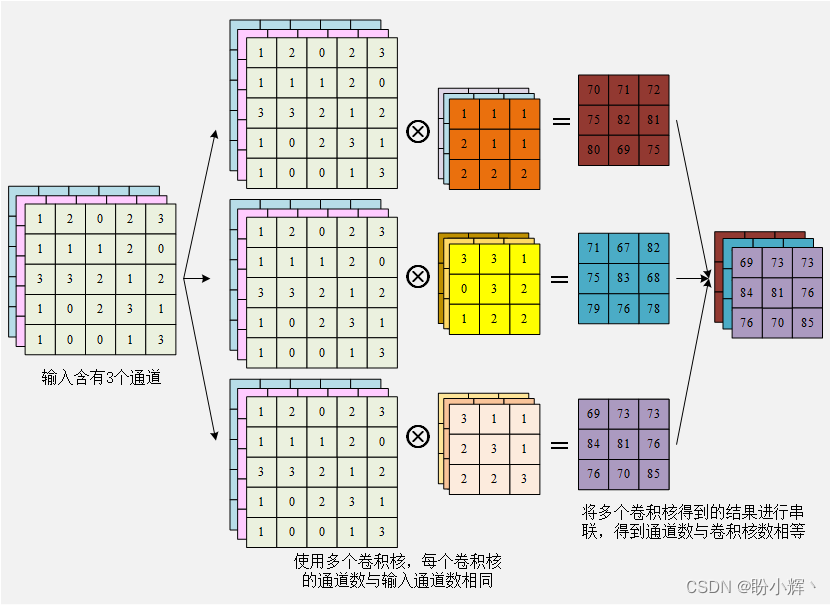2.1.2 步幅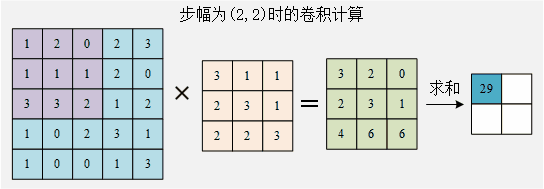2.1.3 填充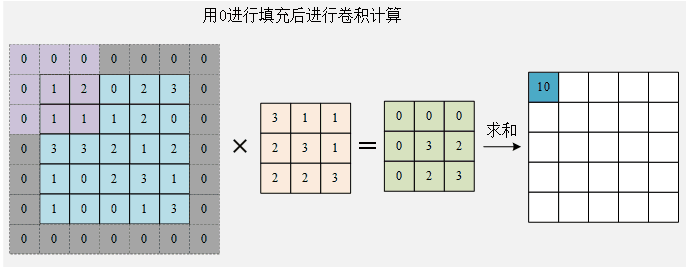2.1.4 激活函數

2.1.5 池化

[ 29 28 20 24 ] \left[ \begin{array}{cc} 29 & 28\\ 20 & 24\\\end{array}\right]

[ 29 28 25 29 20 24 30 26 27 23 26 27 24 25 23 31 ] \left[ \begin{array}{cccc} 29 & 28 & 25 & 29\\ 20 & 24 & 30 & 26\\ 27 & 23 & 26 & 27\\ 24 & 25 & 23 & 31\\\end{array}\right]

[ 29 28 ∣ 25 29 20 24 ∣ 30 26 — — — — — 27 23 ∣ 26 27 24 25 ∣ 23 31 ] = [ 29 30 27 31 ] \left[ \begin{array}{ccccc} 29 & 28 & | & 25 & 29\\ 20 & 24 & | & 30 & 26\\ —&—&—&—&—\\ 27 & 23 & | & 26 & 27\\ 24 & 25 & | & 23 & 31\\\end{array}\right]=\left[ \begin{array}{cc} 29 & 30\\ 27 & 31\\\end{array}\right]

2.2 卷積和池化相比全連接網絡的優勢

3. 使用 Keras 構建卷積神經網絡

3.1 CNN 使用示例

1. 創建輸入和輸出數據集：
import numpy as npx_train = np.array([[[1,2,3,3],                    [2,3,4,5],                    [4,5,6,7],                    [1,3,4,6]],                    [[-1,2,3,-5],                    [2,-2,4,5],                    [-3,5,-4,9],                    [-1,-3,-2,-5]]])y_train = np.array([0, 1])

1. 縮放輸入數據集：
x_train = x_train / 9
1. 對輸入數據集的形狀進行整形，以使每個輸入圖像都以 (寬度 x 高度 x 通道數) 的格式表示：
x_train = x_train.reshape(x_train.shape, x_train.shape, x_train.shape, 1)
1. 準備數據後，構建模型架構，導入相關方法後實例化模型：
from keras.models import Sequentialfrom keras.layers import Conv2D, Flatten, MaxPooling2Dmodel = Sequential()
1. 接下來，我們將執行卷積操作：
model.add(Conv2D(1, (3, 3), input_shape=(4, 4, 1), activation='relu'))

1. 接下來，我們將添加一個執行最大池化操作的網絡層 MaxPooling2D，如下所示：
model.add(MaxPooling2D(pool_size=(2, 2)))

1. 接下來，展平池化層的輸出：
model.add(Flatten())

model.add(Dense(1, activation='sigmoid'))

model.summary()

Model: "sequential"_________________________________________________________________Layer (type)                 Output Shape              Param #   =================================================================conv2d (Conv2D)              (None, 2, 2, 1)           10        _________________________________________________________________max_pooling2d (MaxPooling2D) (None, 1, 1, 1)           0         _________________________________________________________________flatten (Flatten)            (None, 1)                 0         _________________________________________________________________dense (Dense)                (None, 1)                 2         =================================================================Total params: 12Trainable params: 12Non-trainable params: 0_________________________________________________________________

1. 最後，編譯並擬合模型：
model.compile(loss='binary_crossentropy',optimizer='adam',metrics=['acc'])model.fit(x_train, y_train, epochs=50)

3.2 驗證 CNN 輸出

1. 首先，我們提取權重和偏置的相關信息：
print(model.get_weights())

[<tf.Variable 'conv2d/kernel:0' shape=(3, 3, 1, 1) dtype=float32, numpy=array([[[[ 0.1985341 ]],        [[ 0.27599618]],        [[ 0.20717546]]],       [[[-0.41702896]],        [[-0.21289168]],        [[ 0.12980239]]],       [[[-0.14379142]],        [[ 0.55261314]],        [[-0.49706236]]]], dtype=float32)>,<tf.Variable 'conv2d/bias:0' shape=(1,) dtype=float32, numpy=array([0.04198299], dtype=float32)>,<tf.Variable 'dense/kernel:0' shape=(1, 1) dtype=float32, numpy=array([[1.5805249]], dtype=float32)>,<tf.Variable 'dense/bias:0' shape=(1,) dtype=float32, numpy=array([-0.28272304], dtype=float32)>]

1. 使用 modelweights 屬性提取各層的權重值：
print(model.weights)

print(model.predict(x_train.reshape(1,4,4,1)))# 輸出如下# [[0.04299431]]

1. 接下來，我們通過模擬卷積過程進行驗證，對輸入數據執行卷積，輸入圖像的形狀為 4 x 4，而卷積核的形狀為 3 x 3，在代碼中沿著行和列執行矩陣乘法(卷積)：
sumprod = []for i in range(x_train.shape-model.get_weights().shape+1):    for j in range(x_train.shape-model.get_weights().shape+1):        img_subset = np.array(x_train[0,i:(i+3),j:(j+3),0])        filter = model.get_weights().reshape(3,3)        val = np.sum(img_subset*filter) + model.get_weights()        sumprod.append(val)

1. 整形 sumprod 的輸出，以便將其傳遞到池化層：
sumprod = np.array(sumprod).reshape(2, 2, 1)
1. 在卷積的輸出傳遞到池化層之前，先對其使用激活函數：
sumprod = np.where(sumprod>0, sumprod, 0)
1. 將卷積輸出傳遞到池化層，根據以上模型定義，考慮到卷積的輸出為 2 x 2，根據最大池化層的定義我們取輸出中的最大值：
pooling_layer_output = np.max(sumprod)
1. 將池化層的輸出連接到輸出層，將池化層的輸出乘以輸出層中的權重，然後在輸出層中加上偏置值：
intermediate_output_value = pooling_layer_output * model.get_weights() + model.get_weights()
1. 計算 Sigmoid 輸出：
print(1/(1+np.exp(-intermediate_output_value)))

[[0.42994314]]

4. 構建 CNN 模型識別 MNIST 手寫數字 4.1 任務與模型分析

• 輸入形狀為 28 x 28 x 1，使用的卷積核尺寸為 3 x 3 x 1
• 需要註意的是，卷積核的大小可以更改，但是通道數不能更改，需要與輸入通道數相同
• 使用 10 個卷積核
• 輸入圖像經過卷積層後，使用池化層：
• 輸出的圖像尺寸減半
• 展平池化後獲得的輸出
• 展平層連接到一個具有 1000 個單位的隱藏層
• 最後，將隱藏層連接到輸出層，輸出層中有 10 類(包括數字 0-9 )

4.2 CNN 模型構建與訓練

1. 加載並預處理數據：
from keras.layers import Dense,Conv2D,MaxPool2D,Flattenfrom keras.models import Sequentialfrom keras.datasets import mnistfrom keras.utils import np_utilsimport numpy as np(x_train, y_train),(x_test, y_test) = mnist.load_data()x_train = x_train.reshape(x_train.shape, x_train.shape, x_train.shape, 1)x_test = x_test.reshape(x_test.shape, x_test.shape, x_test.shape, 1)x_train = x_train.astype('float32') / 255.x_test = x_test.astype('float32') / 255.y_train = np_utils.to_categorical(y_train)y_test = np_utils.to_categorical(y_test)num_classes = y_test.shape

1. 建立並編譯模型：
model = Sequential()model.add(Conv2D(10, (3, 3), input_shape=(28, 28, 1), activation='relu'))model.add(MaxPool2D(pool_size=(2, 2)))model.add(Flatten())model.add(Dense(512, activation='relu'))model.add(Dense(num_classes, activation='softmax'))model.summary()model.compile(loss='categorical_crossentropy', optimizer='adam', metrics=['acc'])

model.summary()

Model: "sequential"_________________________________________________________________Layer (type)                 Output Shape              Param #   =================================================================conv2d (Conv2D)              (None, 26, 26, 10)        100       _________________________________________________________________max_pooling2d (MaxPooling2D) (None, 13, 13, 10)        0         _________________________________________________________________flatten (Flatten)            (None, 1690)              0         _________________________________________________________________dense (Dense)                (None, 512)               865792    _________________________________________________________________dense_1 (Dense)              (None, 10)                5130      =================================================================Total params: 871,022Trainable params: 871,022Non-trainable params: 0_________________________________________________________________

1. 最後，擬合模型：
model.fit(x_train, y_train,            validation_data=(x_test, y_test),            epochs=10,            batch_size=1024,            verbose=1)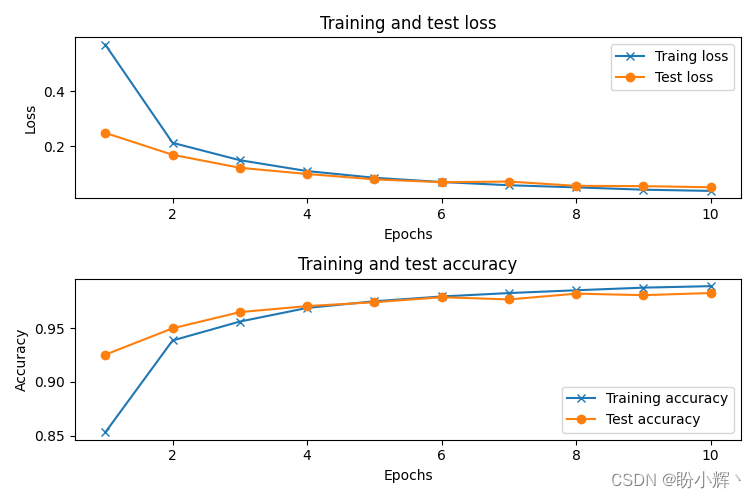4. 接下來，使用所有標簽為 1 的圖像生成均值圖像，並平移 1 個像素：

# 獲取標簽為1的所有圖像輸入x_test1 = x_test[y_test[:, 1]==1]# 利用所有標簽為1的圖像生成均值圖像pic = np.zeros((x_test.shape, x_test.shape))pic2 = np.copy(pic)for i in range(x_test1.shape):    pic2 = x_test1[i, :, :, 0]pic = pic + pic2pic = (pic / x_test1.shape)# 將均值圖像中的每個像素向左平移一個像素for i in range(pic.shape):    if i < 21:        pic[:, i] = pic[:, i+1]# 對平移後的圖像進行預測p = model.predict(pic.reshape(1, x_test.shape, x_test.shape, 1))print(p)c = np.argmax(p)print('CNN預測結果：', c)

[[1.3430370e-05 9.9989212e-01 2.0535077e-05 2.6301734e-07 4.3278211e-05  5.9122913e-06 1.5874346e-05 6.2533190e-06 2.0079199e-06 4.1732173e-07]]CNN預測結果： 1

Keras深度學習實戰（1）——神經網絡基礎與模型訓練過程詳解
Keras深度學習實戰（2）——使用Keras構建神經網絡
Keras深度學習實戰（3）——神經網絡性能優化技術
Keras深度學習實戰（4）——深度學習中常用激活函數和損失函數詳解
Keras深度學習實戰（5）——批歸一化詳解
Keras深度學習實戰（6）——深度學習過擬合問題及解決方法